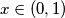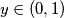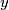### IMO Shortlist 2006 problem N2

Kvaliteta:
Avg: 2,5
Težina:
Avg: 6,0
For$x \in (0, 1)$ let$y \in (0, 1)$ be the number whose$n$-th digit after the decimal point is the$2^{n}$-th digit after the decimal point of$x$. Show that if$x$ is rational then so is$y$.
Izvor: Međunarodna matematička olimpijada, shortlist 2006